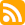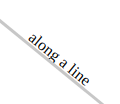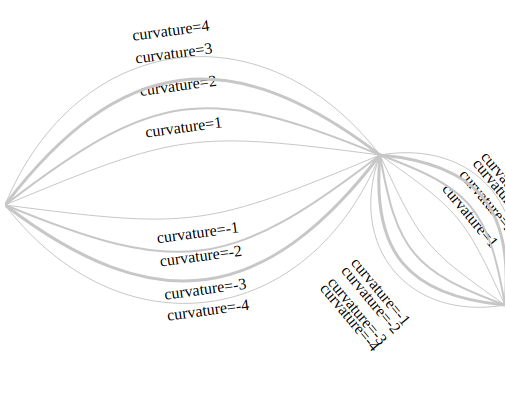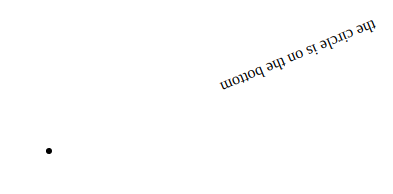#### I'm Learning About: Computing the Shape of Rotated RectanglesJuly2020

It's been a while since I've written one of these. I started working on what turned out to be a pretty major refactoring on my graph rendering project, Yellow, and I've took a detour into some creative work. As the stuff with yellow got deeper and deeper, I kept telling myself that I would write a post when I had something to show for it, and that kept feeling further and further away.

Well, the point of the prompt, I'm Learning About, was to get myself to write more frequently regardless of whether I had something to show for it or not. I've been thinking of changing it toI'm Working On to lower my inhibitions against writing even further, but I find asking myself, "What am I learning right now?" to be consistently challenging, which probably means that it's useful.

I actually started working on this post at Bean There in San Francisco on a Sunday in early March, before quarantine. I had a big vision for what it would accomplish; since it had been so long since my last post, I really needed to make a big splash. There were just a few more technical things I needed to tweak before I was ready to really start working on my next post, I told myself.

Well, as you can see, it's been quite a while since then and I am nowhere near my goal. Those small technical problems tend to explode in complexity as you get more involved in them. I'm currently enmeshed in a geometry problem that came to me out of nowhere while I was working on a feature to render labels along graph edges in yellow. That seemed like a relatively straightforward problem — I already had the ability to render labels at a given position, all I would need to do would be:

• Update the `Label` to accept new props to render itself either above or below the specified position instead centered there; and to render itself with a rotation.
• Add a text `label` prop to the `Line` component, have that component compute the line midpoint and angle, and then render the label there with that rotation.
• Do the same thing for `Curve`, adjusting the point either downward or upwards to account for curvature.

That didn't seem too hard. I could probably do it in a weekend, I told myself.

At first it seemed like things were going well; I could render labels along a line:But I noticed that labels were often mispositioned:In my testing, this problem first manifested with curve labels, so I thought it had something to do with the curve positioning algorithm:

I wasted vast amounts of time tweaking this algorithm, but every time there would be some case that was off. I decided to go back and check simpler cases, which I should have done first:It turns out this problem had nothing to do with the `Curve` code. It came down to the behaior of javascript's getBoundingClientRect method. When called on a rotated element, this method returns to you the bounding rectangle - upright, surrounding the target element:

This is a problem for yellow due to some vagaries with svg rendering - when rendering a label, the position you specify is the top left, not the center like other elements. Since all the simulation math assigns positions based on object centers, I had written some code to automatically determine the size of the rendered element and then use that to determine the svg position (keeping in mind that in svg geometry, up means lower y values):

``export default (ref, callback) => {  let shape;  const intervalId = setInterval(() => {    if (ref.current) {      const rect = ref.current.getBoundingClientRect();      if (        !shape ||        shape.width != rect.width ||        shape.height != rect.height      ) {        shape = { width: rect.width, height: rect.height };        callback(shape);      }    }  }, 10);  return {    stop: () => clearInterval(intervalId)  };}``

So, the `Label` positioning code was moving the element based on the element's width and height, but those values were way too big. As a consequence, label text got displaced by a noticeable amount.

I found some math on the internet that seemed like it made sense. I tried to integrate it into my code, but struggled with some areas, partly due to confusion about the angles that should be used due to the aforementioned y-value quirk in svg geometry. After some false starts, I decided to try to derive the equations myself:

• Start by labeling some things:

`w`, `h`, and `θ` are known. We need to solve for `x` and `y`.

• Draw some triangles:

• Now we should be able to plug and chug into a system of two equations based on trigonometric identities. Working it, I got:

``const widthFormula = (theta, width, height) => {  return (    width + height * Math.cos(theta) * Math.cos(theta)  ) / (    Math.sin(theta) * Math.cos(theta) * Math.cos(theta)  );};const heightFormula = (theta, width, height) => {  const x = widthFormula(theta, width, height);  return (    2 * height * Math.cos(theta) - 2 * x * Math.sin(theta) * Math.cos(theta)  );};``

The math all seemed to work out, but this was giving me insane results: computing values near `0` for `y`, for instance. The rectangles I was getting from `getBoundingClientRect` were near squares, so I decided to try plugging in `Math.PI / 4` for θ. And of course the result came out with a `y` value of `0`.

What was going on? It turns out that this problem doesn't define a single solution, but in fact a family of solutions:

And this is where I stopped and decided to write this post. I need some more information to solve this problem, so I need to do some research to find out if I can get it via javascript. I had had problems using anything other than`getBoundingClientRect`, so I'm not super hopeful.

The thing I found most curious is that the diagram above generates a system of equations that seems solvable, and it seems like you can work the steps to get a specific answer. Did I make a mistake? Somehow I was only solving for the `y=0` case.

Until next time!